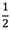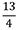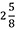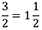## Mathematics 6

### Course Outline

#### Number

• read, write, represent, and describe numbers greater than one million and less than one-thousandth using symbols, expressions, expanded notation, decimal notation, and place-value charts
• explore place value (greater than one million and less than one-thousandth)
• use place-value patterns to understand numbers greater than one million and less than one-thousandth
• compare and order whole numbers and decimal numbers in a variety of ways
• represent, interpret, and model proper fractions (), improper fractions (), and mixed numbers () using concrete materials, pictures, and symbols
• compare and order a set of fractions on a number line
• rename a mixed number as an improper fraction, and an improper fraction as a mixed number ()
• recognize and find factors of numbers (numbers that can be multiplied together to make a specific number) by creating rectangles using tiles or grid paper
• identify the factors of a given number (e.g., the factors of 16 are 1, 2, 4, 8, and 16)
• identify multiples of a given number (e.g., some multiples of 4 are 4, 8, 12, 16, 20, …)
• solve problems involving factors and multiples
• discover the difference between prime and composite numbers and their relationship to factors
• explain why 0 and 1 are neither prime nor composite numbers
• represent ratios concretely, pictorially, and symbolically
• identify and describe ratios
• solve problems involving ratios
• determine equivalent ratios using concrete materials
• understand the relationship between ratio and percent
• represent percent concretely, pictorially, and symbolically
• express a percent as a fraction and as a decimal
• solve percent problems using benchmarks of 25%, 50%, 75%, and 100%
• describe, represent, and record integers (negative numbers like those on a thermometer)
• identify real-life situations in which integers are used
• compare and order integers
• create and solve addition, subtraction, multiplication, and division problems involving whole numbers, and addition and subtraction of decimal numbers
• estimate and calculate sums, differences, products, and quotients of whole numbers using an appropriate method (mental mathematics, paper and pencil, or technology)
• estimate and calculate sums and differences involving decimal numbers using an appropriate strategy (mental mathematics, paper and pencil, or technology)
• represent, concretely, pictorially, and symbolically, the multiplication and division of decimal numbers by a one-digit whole number
• solve and create story problems involving the multiplication and division of decimal numbers by a one-digit whole number
• estimate the products and quotients (answers) involving the multiplication and division of decimal numbers by a one-digit whole number
• use personal strategies to calculate products and quotients (answers) involving the multiplication and division of decimal numbers by a one-digit whole number
• demonstrate and explain why there is a need to have a standard order of operations
• apply the order of operations to solve problems

#### Patterns and Relations

• describe and use pattern rules to continue a pattern and to complete a table of values
• represent pattern rules using an expression
• use pattern rules to describe the relationship within a column and between the columns of a table of values
• identify errors and missing terms within a pattern or table of values
• create tables of values to solve problems
• use a pattern to create a table of values and graph the values
• create a table of values from a pattern or a graph
• describe the relationship shown on a graph
• solve a variety of problems that involve patterns
• use letter variables to show the commutative property of addition and multiplication (e.g., a + b = b + a or a × b = b × a)
• model and explain the preservation of equality using balances and pictures (If I add three cubes to one side of the balance, I must add three cubes to the other side of the balance in order to preserve equality.)
• write equivalent forms of a given equation (3n = 12 is the same as 3n + 5 = 12 + 5 or 2n = 7 is the same as 3(2n) = 3(7)
• solve simple equations that have a missing number or that use a letter to represent the missing number(s) (e.g., 3 × ___ = 12 or 3 × a = 12)
• develop a formula for finding the perimeter of any regular polygon
• develop a formula for finding the area of any rectangle

#### Measurement

• describe angles as a measure of rotation (turn)
• identify and classify angles as acute, obtuse, right, straight, or reflex
• estimate the measure of an angle using a referent
• sketch angles without using a protractor
• measure angles using a protractor
• draw angles using a protractor
• explain, using models, that the sum of the interior angles of any triangle is 180˚
• explain, using models, that the sum of the interior angles of any quadrilateral is 360˚
• explain, using models, how the perimeter of any polygon (2-D shape) can be determined
• generalize a formula for calculating perimeter (distance around the outside)
• explain, using models, how the area (inside space) of any rectangle can be determined
• generalize a formula for calculating area of rectangles
• explain, using models, how the volume (the amount of space occupied by a 3-D object) of any rectangular prism can be determined
• generalize a formula for calculating volume of rectangular prisms
• solve measurement problems that involve perimeter, area, and/or volume

#### Geometry

• sort triangles based on the length of sides (scalene, isosceles, and equilateral) and on the measure of interior angles (acute, obtuse, and right) and explain our sorting rules
• describe triangles according to their side lengths and angle measures
• draw specified triangles
• show that two given triangles are congruent
• sort 2-D shapes as polygons and non-polygons and explain the sorting rule
• sort polygons as regular or irregular and explain the sorting rule
• identify and describe regular and irregular polygons in the environment
• demonstrate congruence (sides to sides; angles to angles) of regular polygons
• show that a 2-D shape and its transformation image (flip, slide, or turn) are congruent
• model, identify, and describe a combination of transformations (flips, slides, or turns) on 2-D shapes
• predict or draw what happens when students combine transformations
• analyze a design and describe the original shape and the transformations used to create this design
• create a design using transformations
• describe why a shape will or will not tessellate (create a tiling pattern with no gaps or overlap)
• create and describe tessellations
• find examples of tessellations in the real world
• plot points on the first quadrant of the Cartesian plane using ordered pairs
• match points on the first quadrant of the Cartesian plane with the corresponding ordered pairs
• draw shapes/designs on the first quadrant of the Cartesian plane when given ordered pairs
• create shapes/designs on the first quadrant of the Cartesian plane and identify the ordered pairs used to create them
• identify the ordered pairs of the vertices (corners) of any 2-D shape placed on the first quadrant of the Cartesian plane
• perform transformations (flips, slides, and turns) on the first quadrant of the Cartesian plane and identify the ordered pairs of the original shape and its image
• describe the change in the position of the vertices of a 2-D shape after a transformation has occurred

#### Statistics and Probability

• read and interpret line graphs
• create line graphs from a table of values or a set of data
• explain the difference between discrete data (data that has finite values and can be counted, such as the number of pets) and continuous data (data that includes an infinite number of values between two points, such as plant growth over time)
• determine whether a set of data is continuous and can be represented with a line graph or is discrete and can be represented by a series of points
• determine the most appropriate type of graph (pictographs, line plots, bar graphs, double bar graphs, and line graphs) for displaying a set of data
• solve problems by graphing data and interpreting graphs
• select an appropriate method for collecting data to answer a question
• design and use questionnaires for collecting data and answering questions
• identify various ways that data can be collected
• explain when it is appropriate to use a database to collect data
• gather data using electronic media
• make observations about everyday probability situations
• identify the possible outcomes for a given probability experiment
• predict and determine the theoretical probability of a certain outcome occurring
• design and conduct probability experiments and analyze the information that is collected
• explain the difference between experimental probability and theoretical probability
• compare experimental probability with theoretical probability from a probability experiment
• explain that as the number of trials in a probability experiment increases, the experimental probability approaches theoretical probability for an outcome

Updated January 31, 2022